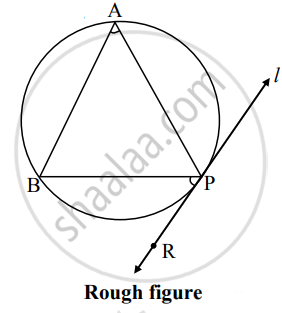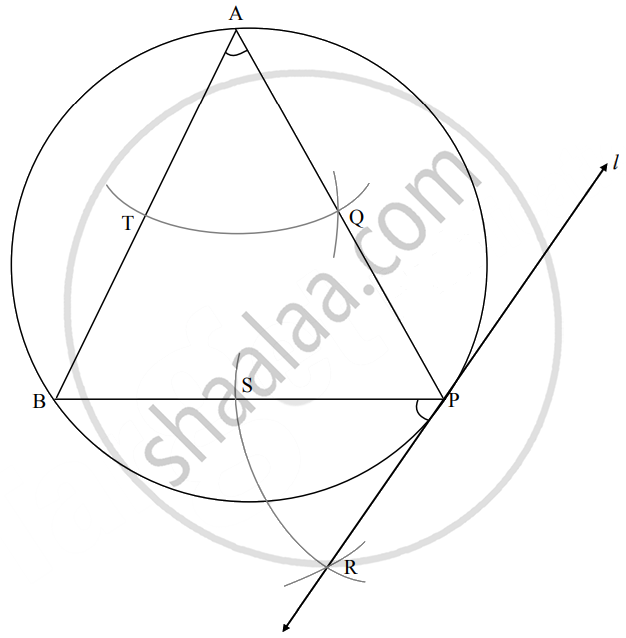# Draw a circle of radius 4.2 cm. Draw a tangent to the circle at point P on the circle without using the center of the circle - Geometry

Diagram

Draw a circle of radius 4.2 cm. Draw a tangent to the circle at point P on the circle without using the center of the circle

#### SolutionSteps of construction:

1. Draw a circle of radius 4.2 cm and take any point P on it.
2. Draw chord BP of any length and an inscribed ∠BAP of any measure.
3. By taking A as a centre and any convenient distance on compass draw an arc intersecting the arms of ∠BAP in points P and Q.
4. With P as a centre and the same distance in the compass, draw an arc intersecting the chord BP at point S.
5. Taking radius equal to TQ and S as a centre, draw an arc intersecting the previously drawn arc. Name the point of intersection as R.
6. Draw line RP.
Line RP is the required tangent to the circle.
Concept: Construction of a Tangent to the Circle at a Point on the Circle
Is there an error in this question or solution?

Share